## boxplot on a formula:
boxplot(count ~ spray, data = InsectSprays, col = "lightgray")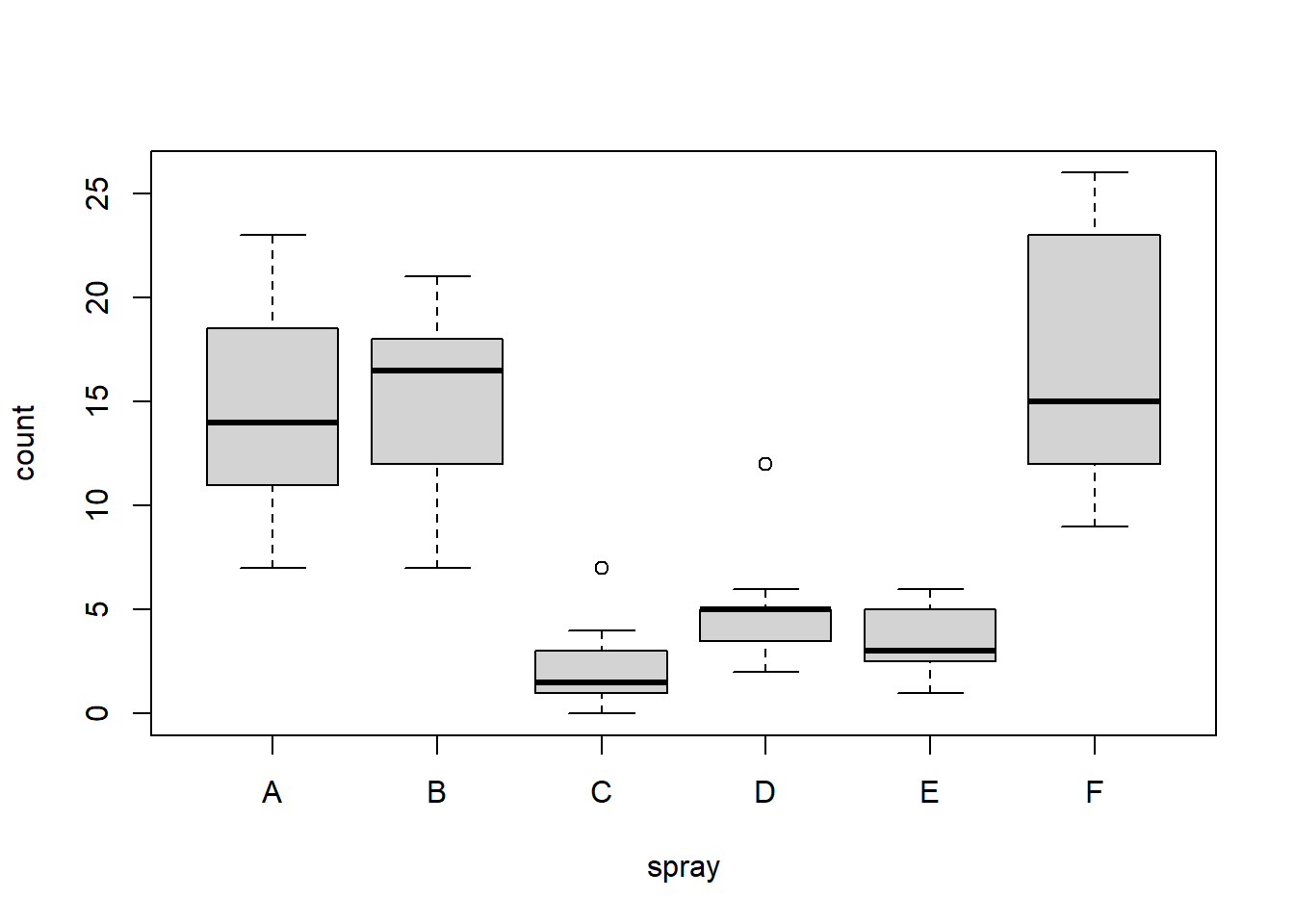# *add* notches (somewhat funny here <--> warning "notches .. outside hinges"):
boxplot(count ~ spray, data = InsectSprays, col = "lightgray")
boxplot(count ~ spray, data = InsectSprays,
notch = TRUE, add = TRUE, col = "blue")
## Warning in bxp(list(stats = structure(c(7, 11, 14, 18.5, 23, 7, 12, 16.5, :
## some notches went outside hinges ('box'): maybe set notch=FALSE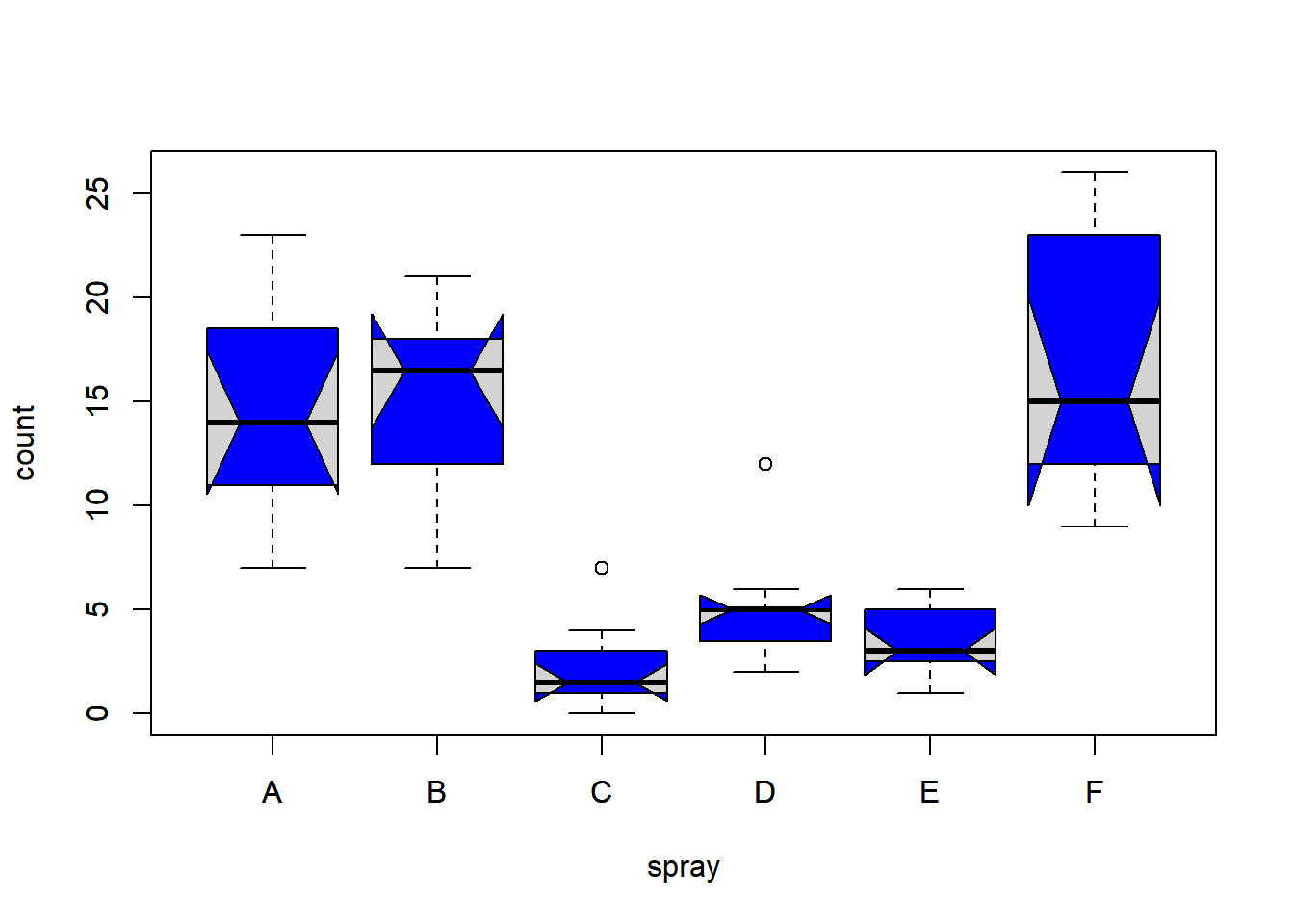boxplot(decrease ~ treatment, data = OrchardSprays, col = "bisque",
log = "y")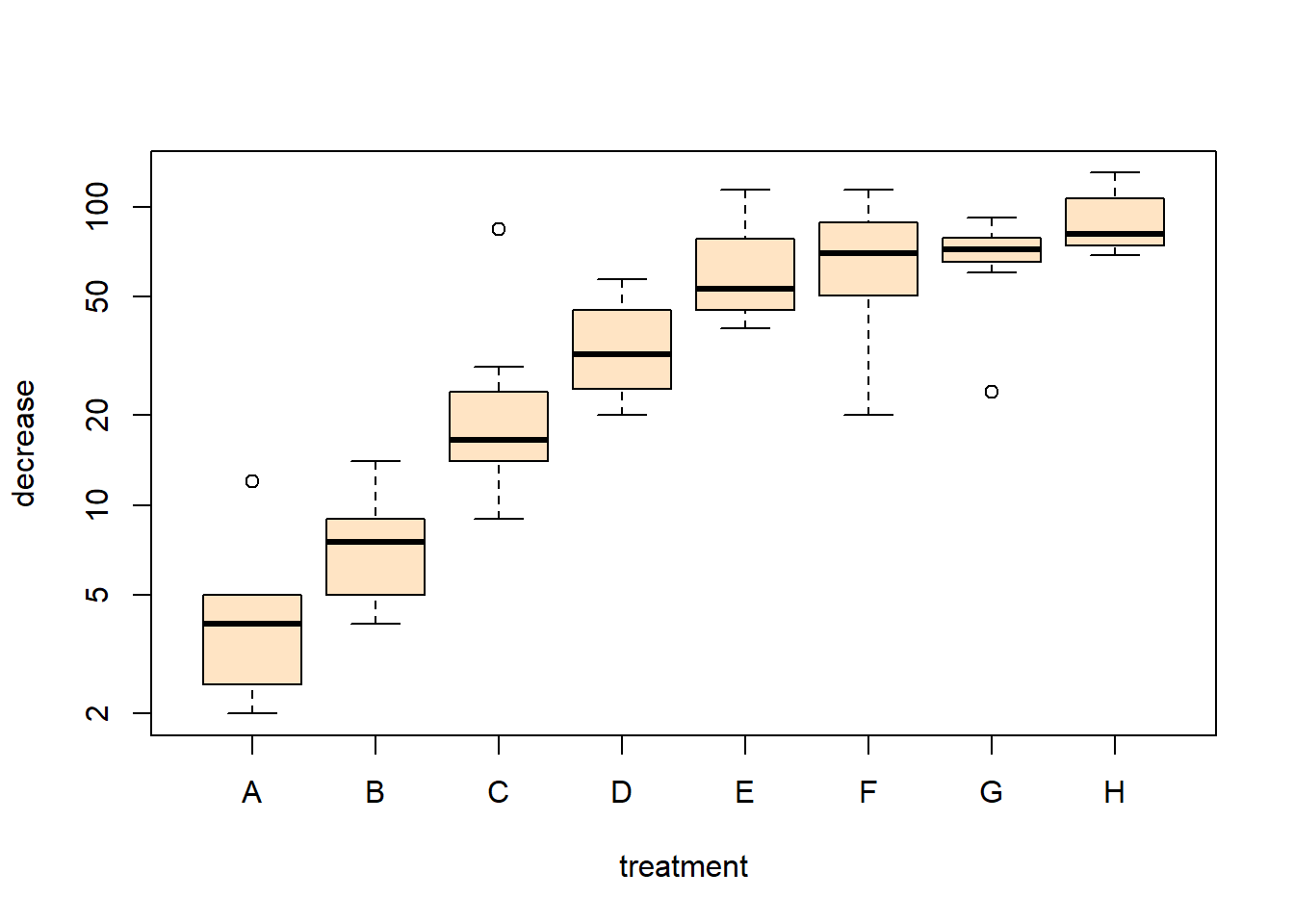## horizontal=TRUE, switching  y <--> x :
boxplot(decrease ~ treatment, data = OrchardSprays, col = "bisque",
log = "x", horizontal=TRUE)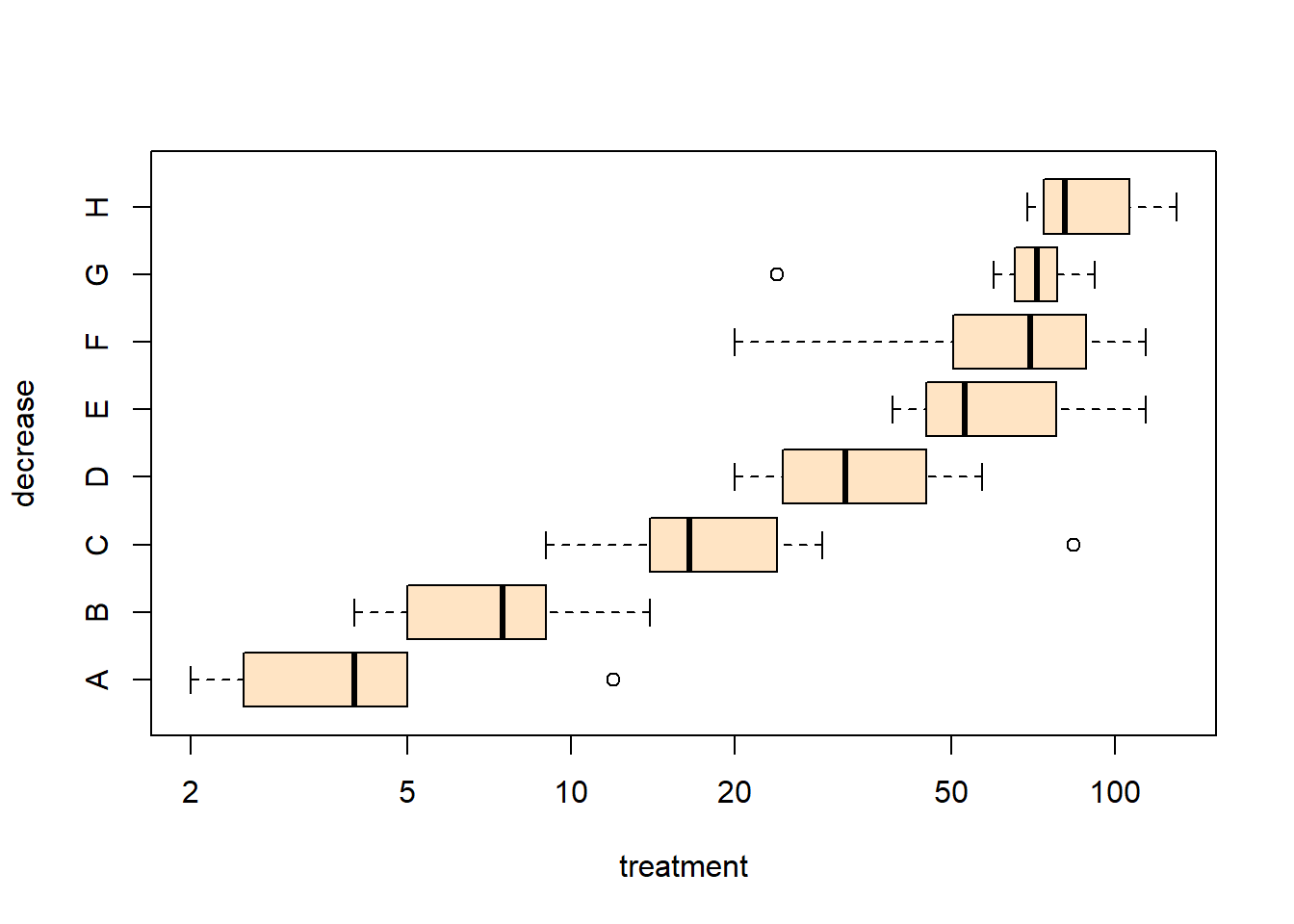rb <- boxplot(decrease ~ treatment, data = OrchardSprays, col = "bisque")
title("Comparing boxplot()s and non-robust mean +/- SD")
mn.t <- tapply(OrchardSprays$decrease, OrchardSprays$treatment, mean)
sd.t <- tapply(OrchardSprays$decrease, OrchardSprays$treatment, sd)
xi <- 0.3 + seq(rb$n) points(xi, mn.t, col = "orange", pch = 18) arrows(xi, mn.t - sd.t, xi, mn.t + sd.t, code = 3, col = "pink", angle = 75, length = .1)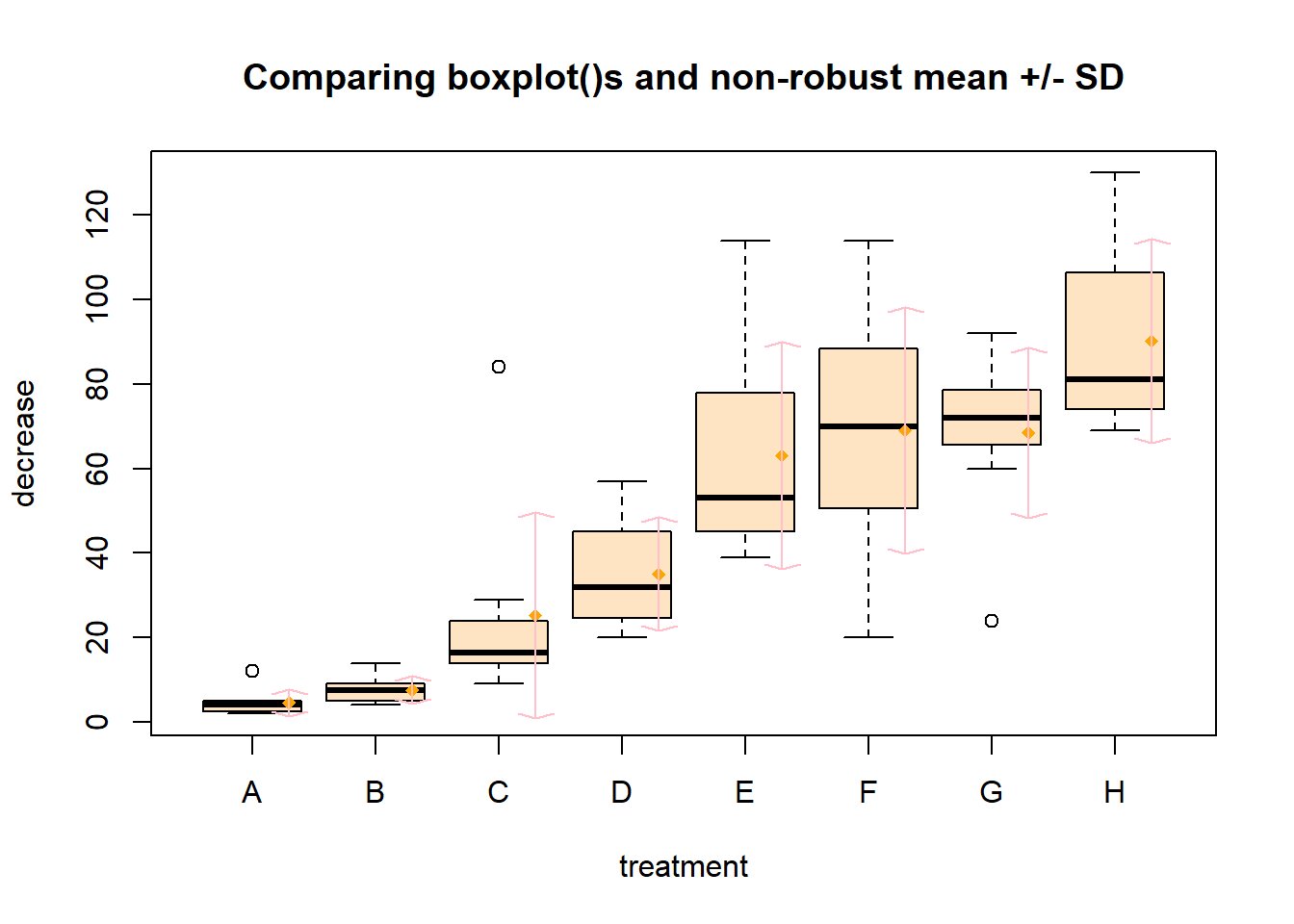## boxplot on a matrix: mat <- cbind(Uni05 = (1:100)/21, Norm = rnorm(100), 5T = rt(100, df = 5), Gam2 = rgamma(100, shape = 2)) boxplot(mat) # directly, calling boxplot.matrix()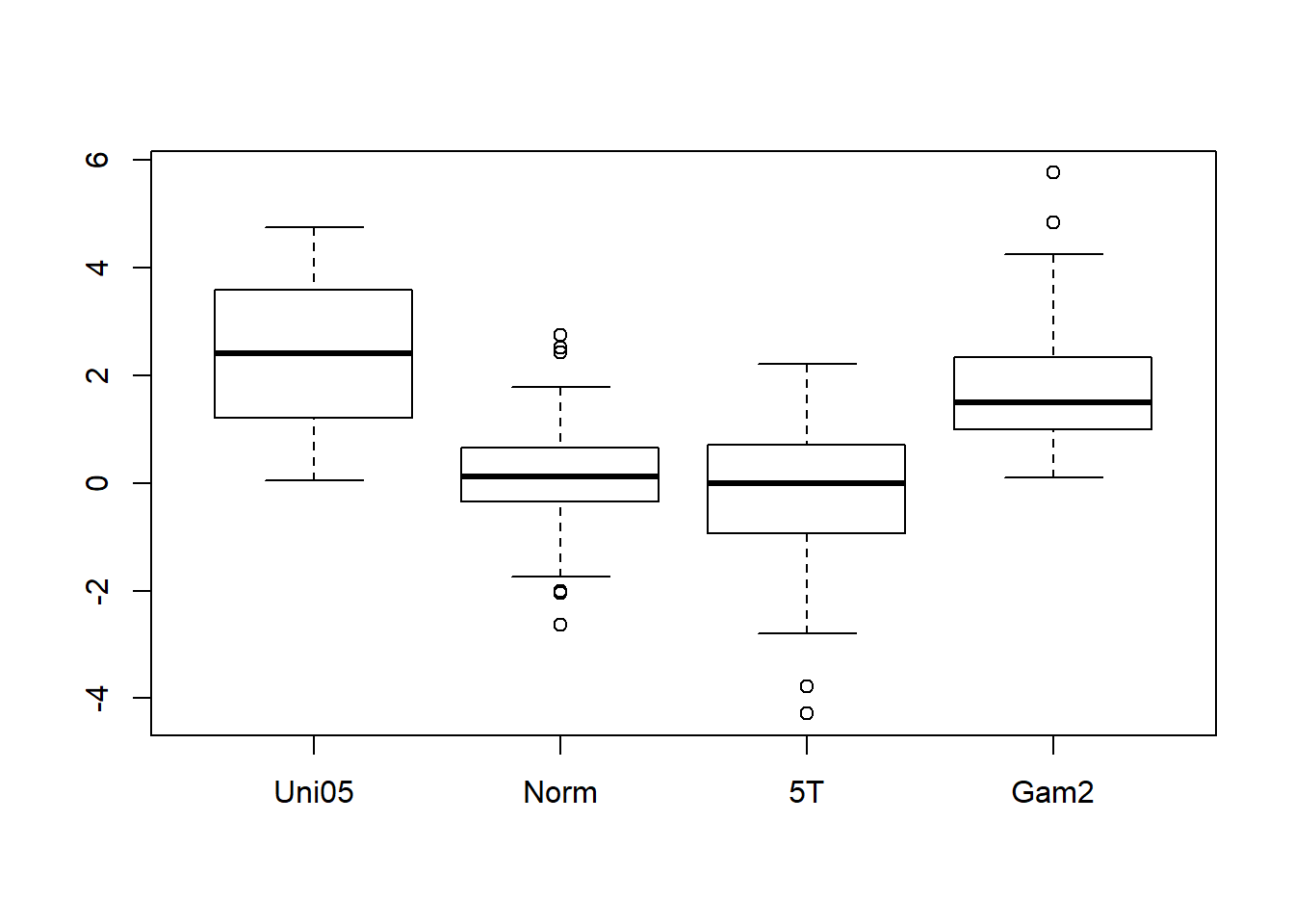## boxplot on a data frame: df. <- as.data.frame(mat) par(las = 1) # all axis labels horizontal boxplot(df., main = "boxplot(*, horizontal = TRUE)", horizontal = TRUE)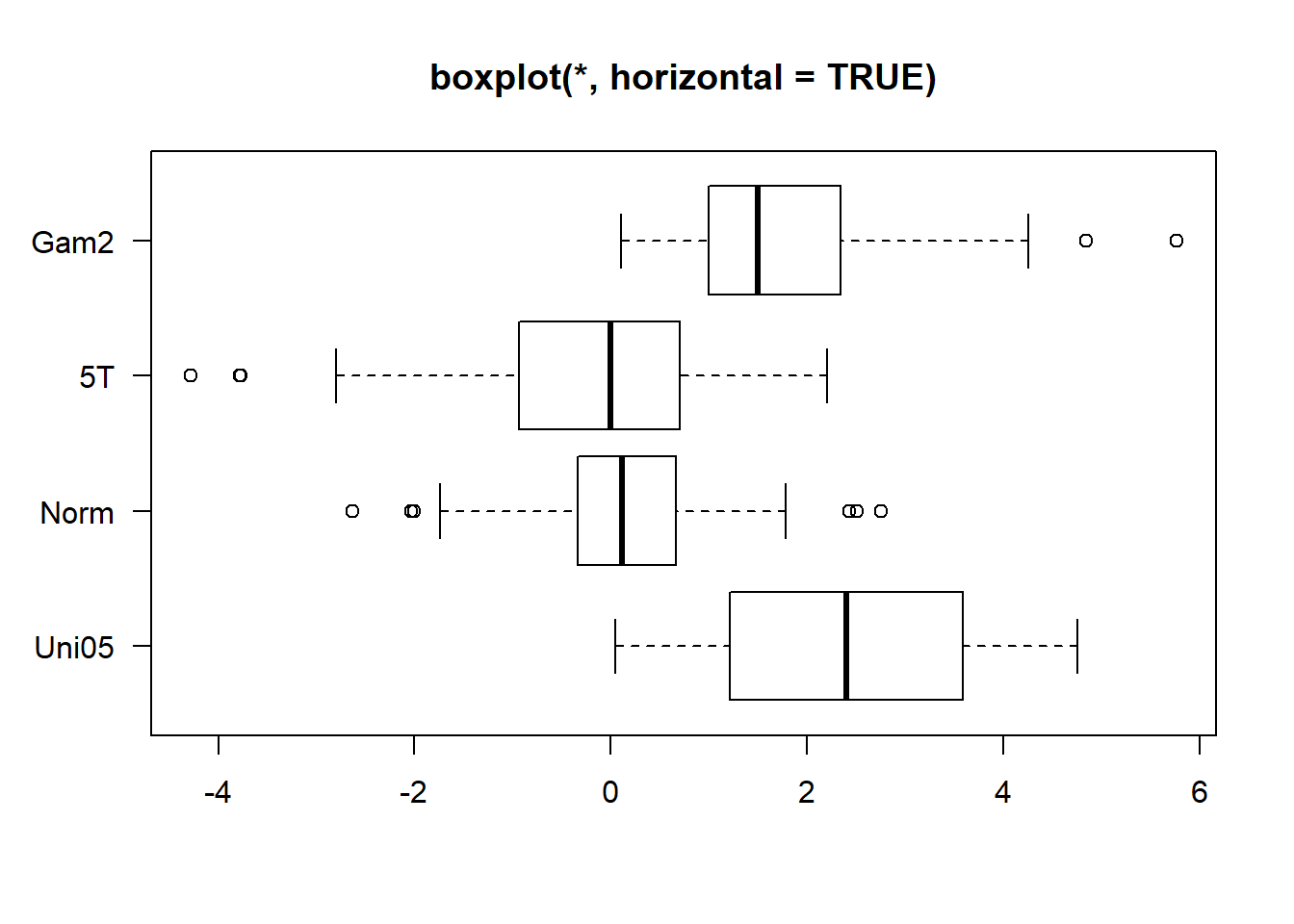## Using 'at = ' and adding boxplots -- example idea by Roger Bivand : boxplot(len ~ dose, data = ToothGrowth, boxwex = 0.25, at = 1:3 - 0.2, subset = supp == "VC", col = "yellow", main = "Guinea Pigs' Tooth Growth", xlab = "Vitamin C dose mg", ylab = "tooth length", xlim = c(0.5, 3.5), ylim = c(0, 35), yaxs = "i")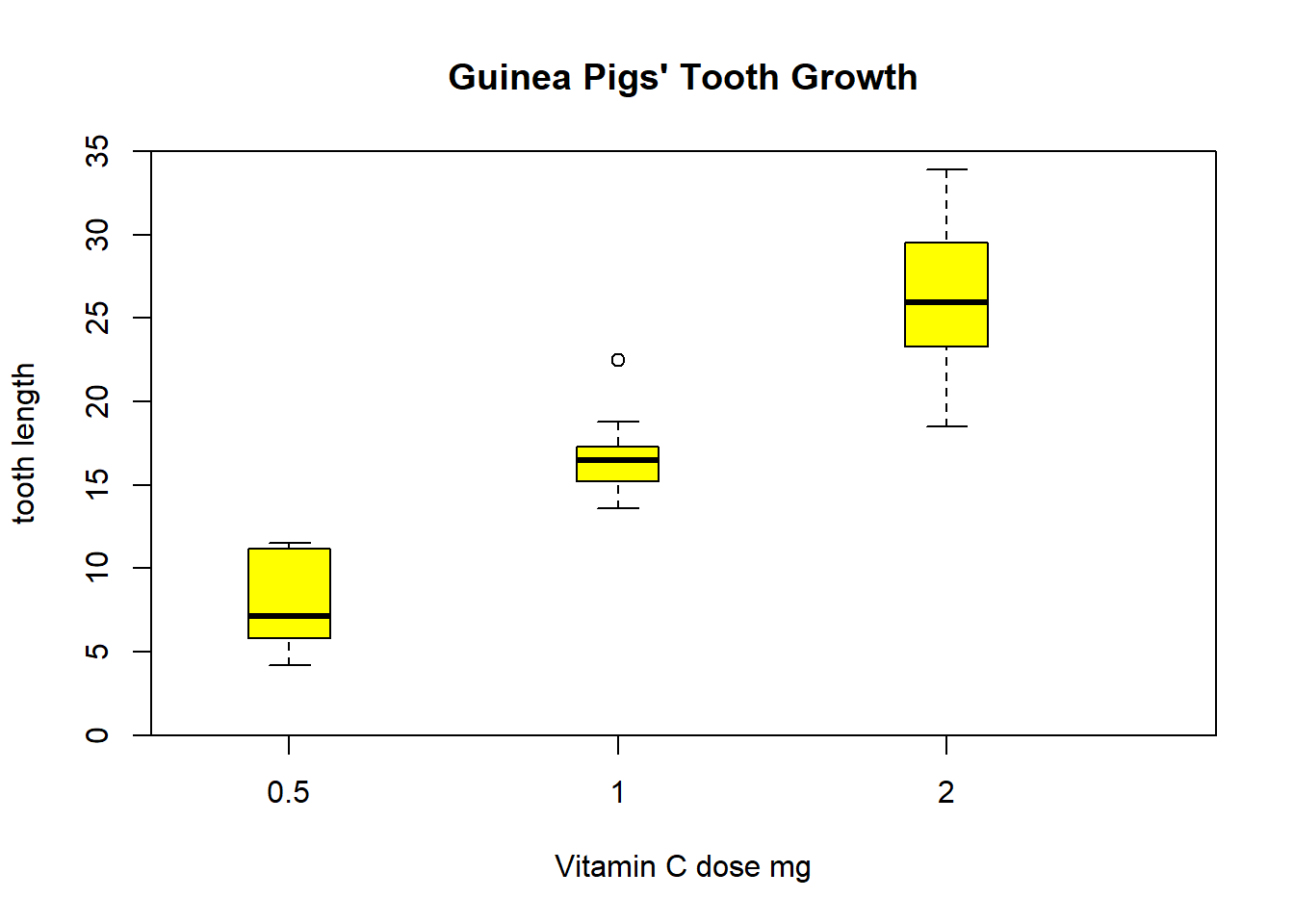## Using 'at = ' and adding boxplots -- example idea by Roger Bivand : boxplot(len ~ dose, data = ToothGrowth, boxwex = 0.25, at = 1:3 - 0.2, subset = supp == "VC", col = "yellow", main = "Guinea Pigs' Tooth Growth", xlab = "Vitamin C dose mg", ylab = "tooth length", xlim = c(0.5, 3.5), ylim = c(0, 35), yaxs = "i") boxplot(len ~ dose, data = ToothGrowth, add = TRUE, boxwex = 0.25, at = 1:3 + 0.2, subset = supp == "OJ", col = "orange") legend(2, 9, c("Ascorbic acid", "Orange juice"), fill = c("yellow", "orange"))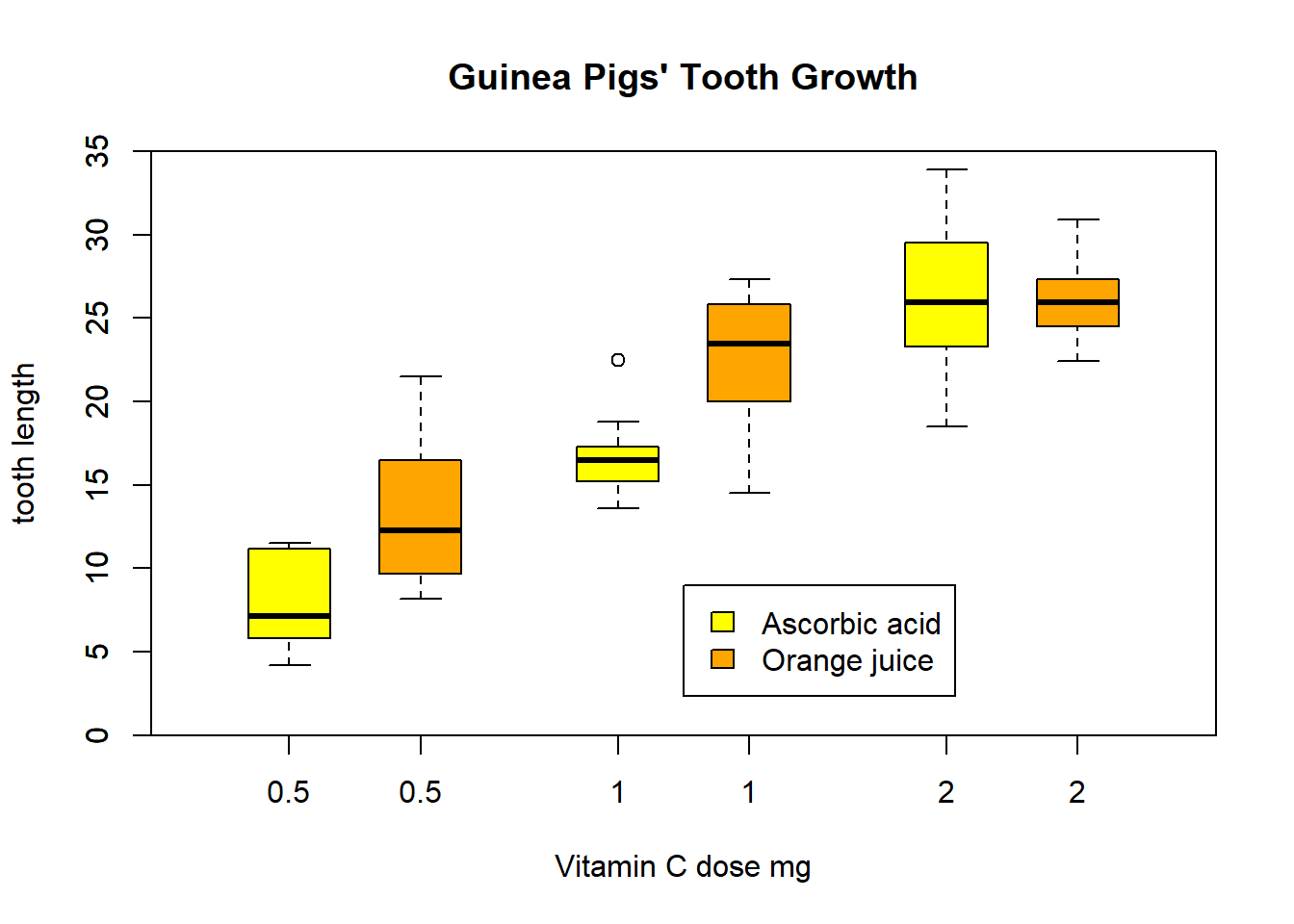## With less effort (slightly different) using factor *interaction*: boxplot(len ~ dose:supp, data = ToothGrowth, boxwex = 0.5, col = c("orange", "yellow"), main = "Guinea Pigs' Tooth Growth", xlab = "Vitamin C dose mg", ylab = "tooth length", sep = ":", lex.order = TRUE, ylim = c(0, 35), yaxs = "i")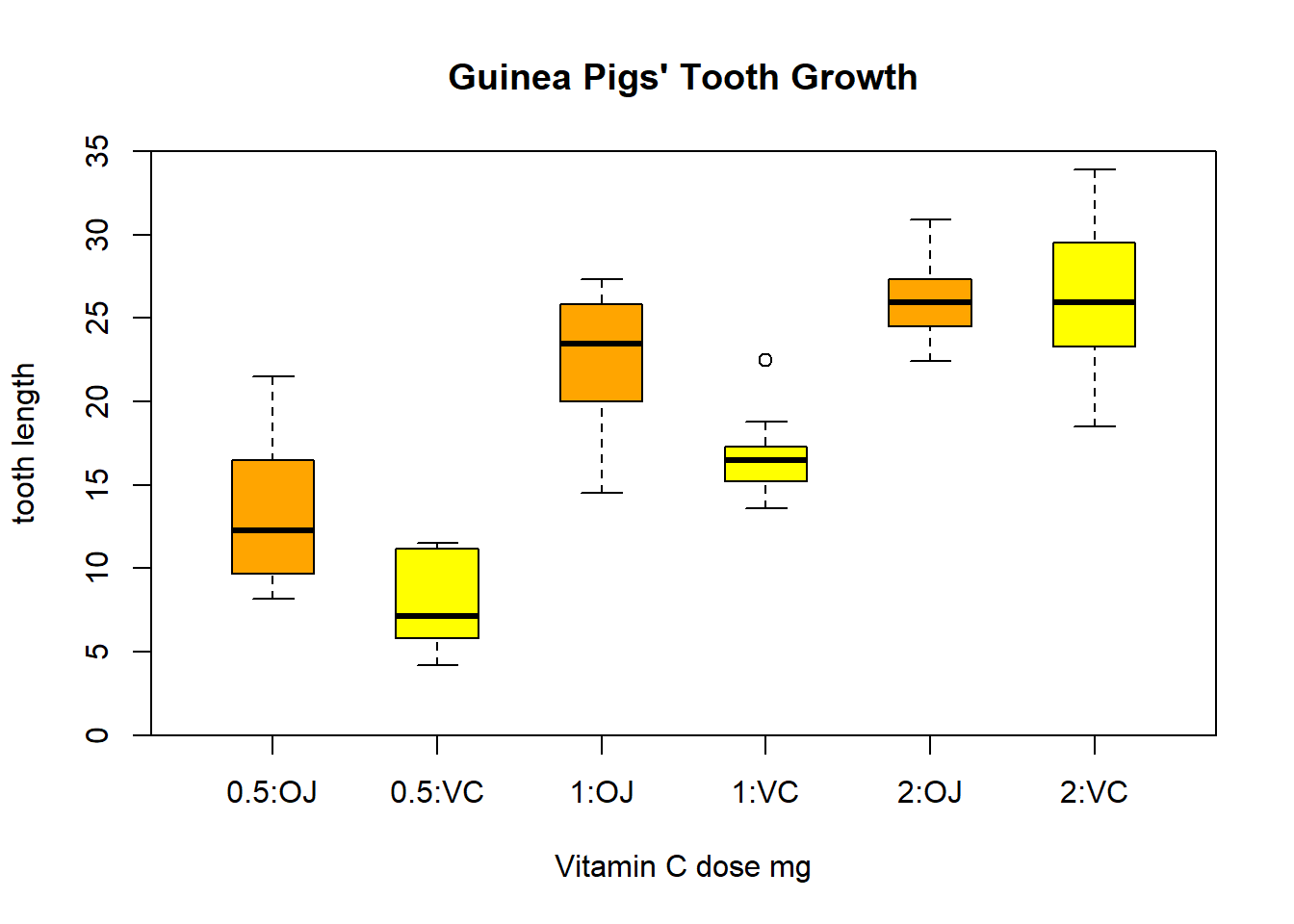boxplot(mpg ~ cyl, data = mtcars, col = "lightgray", varwidth = TRUE, main = "mpg vs cylinders", ylab = "mpg",xlab = "cylinders")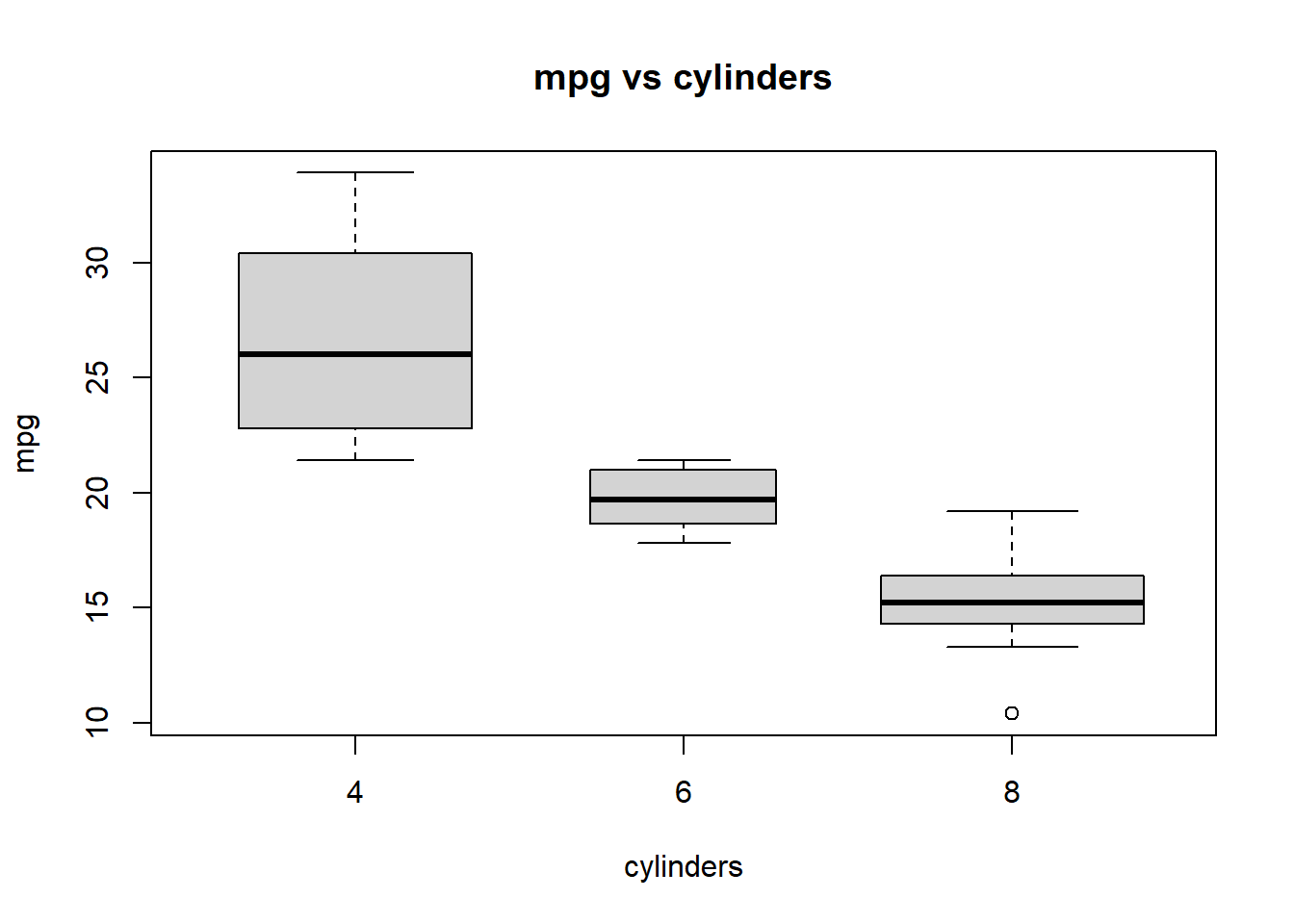fivenum(mtcars$mpg) # the numbers used to create the boxplot
##  10.40 15.35 19.20 22.80 33.90